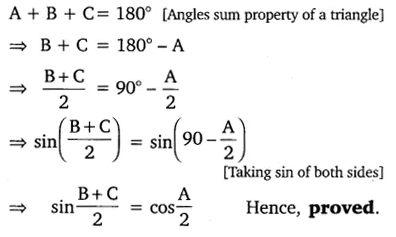# If A, B and C are interior angles of a triangle ABC, then show that:

If A, B and C are interior angles of a triangle ABC, then show that: sin ($\frac { A }{ 2 }$) = cos$\frac { A }{ 2 }$.